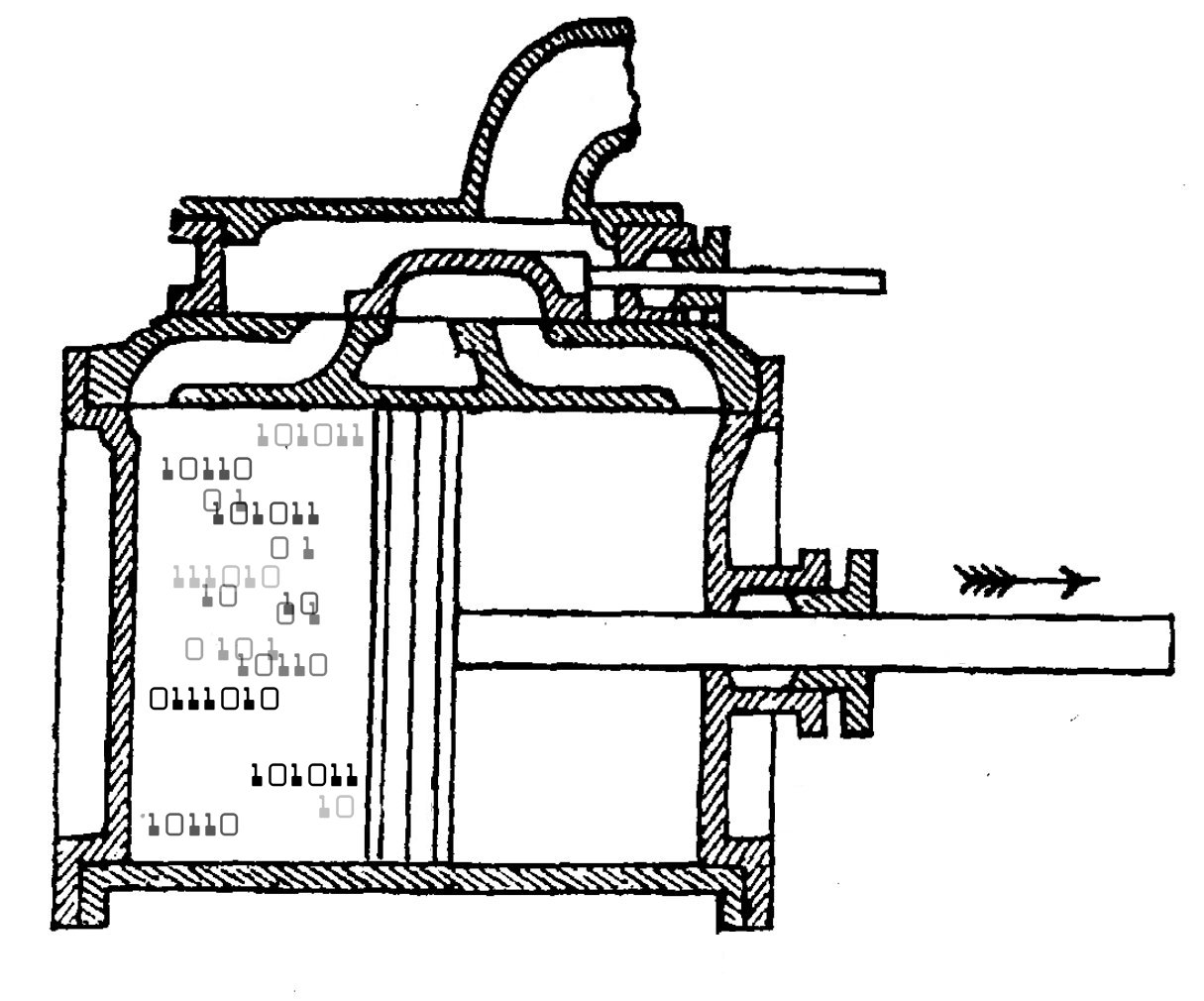## Computation and ThermodynamicsThis talk is about the link between computation and entropy. I take the idea of a Turing machine for granted, but starting with that I explain recursive functions, the Church-Turing thesis, Kolomogorov complexity, the relation between Kolmogorov complexity and Shannon entropy, the uncomputability of Kolmogorov complexity, the 'complexity barrier', Levin's computable version of complexity, and finally my work with Mike Stay on algorithmic thermodynamics.

You can see the slides here. For more details, read this:

In my talk slides I mention the 'complexity barrier', and state this theorem:

Theorem. Choose your favorite set of axioms for math. If it's finite and consistent, there exists C > 0, the 'complexity barrier', such that for no natural number n can you prove the Kolmogorov complexity of n exceeds C.

For a sketch of the proof of this result, go here:

In my talk I showed a movie related to this: an animated video created in 2009 using a program less than 4 kilobytes long that runs on a Windows XP machine:

For more, try these blog articles: